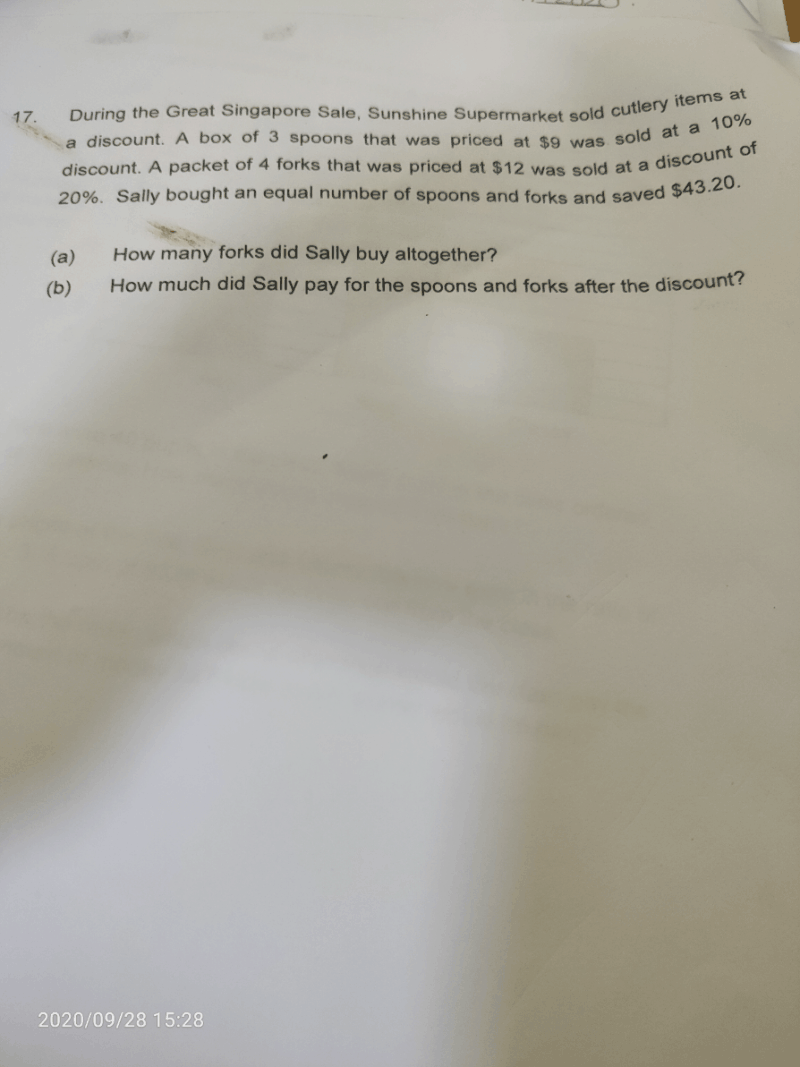# QuestionBox of 3 spoons, original = \$9

Box of 3 spoons, discounted price = (100% – 10%) x \$9 = \$8.10

Packet of 4 forks, original = \$12

Packet of 4 forks, discounted price = (100% – 20%) x \$12 = \$9.60

LCM of 3 and 4 —> 12

1 set, original = 12 spoons and 12 forks (24 cutlery items) = (12 ÷ 3) x \$9 + (12 ÷ 4) x \$12 = \$72

1 set, discounted price = 12 spoons and 12 forks (24 cutlery items) = (12 ÷ 3) x \$8.10 + (12 ÷ 4) x \$9.60 = \$61.20

Diff in both sets (saved) = \$72 – \$61.20 = \$10.80

No. of sets = \$43.20 ÷ \$10.80 = 4

Total forks Sally bought = 4 x 4 = 16 (a)

Total paid, discounted price = 4 x \$61.20 = \$244.80 (b)

Hope you understand and all the best for your exams! :))

2 Replies 1 Like

May i know if the answer for a) should be 4*12 forks =48

0 Replies 0 Likes

Thank you Suma for helping

0 Replies 0 Likes